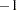The LIFEREG Procedure
 OUTEST= Data Set

The OUTEST= data set contains parameter estimates and the log likelihood for the model. You can specify a label in the MODEL statement to distinguish between the estimates for different models fit with the LIFEREG procedure. If the COVOUT option is specified, the OUTEST= data set also contains the estimated covariance matrix of the parameter estimates. Note that, if the LIFEREG procedure does not converge, the parameter estimates are set to missing in the OUTEST data set.

The OUTEST= data set contains all variables specified in the MODEL statement and the BY statement. One observation consists of parameter values for the model with the dependent variable having the value. If the COVOUT option is specified, there are additional observations containing the rows of the estimated covariance matrix. For these observations, the dependent variable contains the parameter estimate for the corresponding row variable. The following variables are also added to the data set:

_MODEL_

a character variable containing the label of the MODEL statement, if present. Otherwise, the variable’s value is blank.

_NAME_

a character variable containing the name of the dependent variable for the parameter estimates observations or the name of the row for the covariance matrix estimates

_TYPE_

a character variable containing the type of the observation, either PARMS for parameter estimates or COV for covariance estimates

_DIST_

a character variable containing the name of the distribution modeled

_LNLIKE_

a numeric variable containing the last computed value of the log likelihood

INTERCEPT

a numeric variable containing the intercept parameter estimates and covariances

_SCALE_

a numeric variable containing the scale parameter estimates and covariances

_SHAPE1_

a numeric variable containing the first shape parameter estimates and covariances if the specified distribution has additional shape parameters

Any BY variables specified are also added to the OUTEST= data set.Previous Page | Next Page | Top of Page